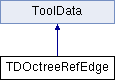cgma
TDOctreeRefEdge Class Reference

`#include <TDOctreeRefEdge.hpp>`

Inheritance diagram for TDOctreeRefEdge:List of all members.

## Public Member Functions

TDOctreeRefEdge ()
~TDOctreeRefEdge ()
CubitBoolean get_visit (void)
void set_visit (CubitBoolean type)
void clean_gpoint_list (void)
void set_mref_edge (RefEdge *ptr_mref_edge)
int get_gpoint_list_length (void)
CubitBoolean generate_gpoint_list (double decimation_ang)

## Static Public Member Functions

static int is_td_skl_mref_edge (const ToolData *td)
static TDOctreeRefEdgeget_td (RefEdge *mref_edge)
static void decimate_curve_points_for_source_entity (GMem &point_data, double angle_tolerance, GMem &result_point_data)
static CubitBoolean find_curve_curvature_using_three_points (CubitVector point_a, CubitVector point_b, CubitVector point_c, CubitVector &curvature)

## Private Attributes

GMemresultPointData
RefEdgerefEdge
CubitBoolean visit

## Detailed Description

Definition at line 21 of file TDOctreeRefEdge.hpp.

## Constructor & Destructor Documentation

 TDOctreeRefEdge::TDOctreeRefEdge ( )

Definition at line 18 of file TDOctreeRefEdge.cpp.

```                                {
resultPointData = new GMem;
visit = CUBIT_FALSE;
}
```
 TDOctreeRefEdge::~TDOctreeRefEdge ( )

Definition at line 25 of file TDOctreeRefEdge.cpp.

```                                 {
clean_gpoint_list();
//PRINT_INFO("Inside ~TDOctreeRefEdge\n");
}
```

## Member Function Documentation

 CubitStatus TDOctreeRefEdge::add_td ( RefEdge * mref_edge ) ` [static]`

Definition at line 43 of file TDOctreeRefEdge.cpp.

```{
ToolData *td;
td = mref_edge->get_TD(&TDOctreeRefEdge::is_td_skl_mref_edge);
if ( td == NULL )
{
TDOctreeRefEdge *td_gm = new TDOctreeRefEdge;
td_gm->set_mref_edge( mref_edge );
}
else
{
TDOctreeRefEdge *td_gm = CAST_TO(td, TDOctreeRefEdge);
td_gm->set_mref_edge( mref_edge );
}
return CUBIT_SUCCESS;
}
```
 void TDOctreeRefEdge::clean_gpoint_list ( void )

Definition at line 30 of file TDOctreeRefEdge.cpp.

```                                       {
delete resultPointData;
}
```
 void TDOctreeRefEdge::decimate_curve_points_for_source_entity ( GMem & point_data, double angle_tolerance, GMem & result_point_data ) ` [static]`

Definition at line 85 of file TDOctreeRefEdge.cpp.

```{
// pointListCount instead of point_data.point_list_size()  (they are different)
int num_pre_points = point_data.pointListCount;

// only decimate if we have more than 2 points
if(num_pre_points <= 2)
{
result_point_data = point_data;
return;
}

// get threshold value
const double threshold = cos( DEGREES_TO_RADIANS(angle_tolerance) );

// mark array for what to keep and throw
char* mark_array = new char[num_pre_points];
// zero means keep the point, non-zero means remove the point
memset(mark_array, 0, num_pre_points);

int num_points_to_keep = 2;   // initialize to keep start and end points

int i;
GPoint* point_data_array = point_data.point_list();
// initialize first point
GPoint* pre_point = &(point_data_array);
// traverse all but start and end points
for(i=1; i<(num_pre_points-1); i++)
{

// create two vectors
// one from i-1 to i and another from i to i+1
GPoint* point = &(point_data_array[i]);
GPoint* next_point = &(point_data_array[i+1]);

CubitVector v1(CubitVector(pre_point->x, pre_point->y, pre_point->z),
CubitVector(point->x, point->y, point->z));
v1.normalize();

CubitVector v2(CubitVector(point->x, point->y, point->z),
CubitVector(next_point->x, next_point->y, next_point->z));
v2.normalize();

// compute whether to keep the point
double dot_product = v1 % v2;

bool keep = dot_product > threshold ? false : true;

// if keep
if(keep)
{
num_points_to_keep++;
pre_point = point;
}
// if not keep
else
{
mark_array[i] = 1;
}
}

// make a new GPoint array
GPoint* new_point_list = new GPoint[num_points_to_keep];
int new_point_list_count = 0;
// copy points to keep into the new array
for(i=0; i<num_pre_points; i++)
{
if(mark_array[i] == 0)
{
new_point_list[new_point_list_count] = point_data_array[i];
new_point_list_count++;
}
}

delete [] mark_array;

assert(new_point_list_count == num_points_to_keep);
//printf("reduced points from %i to %i\n", num_pre_points, num_points_to_keep);

// put data into the result gmem
result_point_data.replace_point_list(new_point_list, new_point_list_count, new_point_list_count);
result_point_data.points_consolidated(CUBIT_TRUE);
}
```
 CubitBoolean TDOctreeRefEdge::find_curve_curvature_using_three_points ( CubitVector point_a, CubitVector point_b, CubitVector point_c, CubitVector & curvature ) ` [static]`

Definition at line 189 of file TDOctreeRefEdge.cpp.

```                                                                                                                                                           {

CubitVector vec_ba, vec_bc;

vec_ba = point_a - point_b;
vec_bc = point_c - point_b;

// Squares of lengths of the edges incident to `a'.
double ba_length = vec_ba.length_squared();
double bc_length = vec_bc.length_squared();

// Cross product of these edges.
// (Take your chances with floating-point roundoff.)
CubitVector cross = vec_ba * vec_bc;

// Calculate the denominator of the formulae.
double denominator = 0.5 / (cross % cross);
assert(denominator != 0.0);

// Calculate offset (from `a') of circumcenter.
CubitVector circle_center  = (ba_length * vec_bc - bc_length * vec_ba) * cross;
circle_center *= denominator;

circle_center.normalize();

curvature = circle_center;
return CUBIT_TRUE;
}
```
 CubitBoolean TDOctreeRefEdge::generate_gpoint_list ( double decimation_ang )

Definition at line 169 of file TDOctreeRefEdge.cpp.

```{
// get facets from cgm
GMem point_data;
refEdge->get_graphics( point_data );
// decimate the facets to the resolution we want

TDOctreeRefEdge::decimate_curve_points_for_source_entity( point_data, decimation_ang, *resultPointData);

return CUBIT_TRUE;
}
```
 int TDOctreeRefEdge::get_gpoint_list_length ( void ) ` [inline]`

Definition at line 51 of file TDOctreeRefEdge.hpp.

```{ return resultPointData->pointListCount; }
```
 GPoint* TDOctreeRefEdge::get_head_gpoint ( ) ` [inline]`

Definition at line 49 of file TDOctreeRefEdge.hpp.

```{ return resultPointData->point_list(); }
```
 TDOctreeRefEdge * TDOctreeRefEdge::get_td ( RefEdge * mref_edge ) ` [static]`

Definition at line 70 of file TDOctreeRefEdge.cpp.

```{
ToolData *td;
td = mref_edge->get_TD(&TDOctreeRefEdge::is_td_skl_mref_edge);
if ( td != NULL )
{
TDOctreeRefEdge *td_gm = CAST_TO(td, TDOctreeRefEdge);
return td_gm;
}
return (TDOctreeRefEdge*) NULL;
}
```
 CubitBoolean TDOctreeRefEdge::get_visit ( void ) ` [inline]`

Definition at line 39 of file TDOctreeRefEdge.hpp.

```{ return visit; }
```
 static int TDOctreeRefEdge::is_td_skl_mref_edge ( const ToolData * td ) ` [inline, static]`

Definition at line 47 of file TDOctreeRefEdge.hpp.

```{return (CAST_TO(const_cast<ToolData*>(td), TDOctreeRefEdge) != NULL);}
```
 void TDOctreeRefEdge::set_mref_edge ( RefEdge * ptr_mref_edge ) ` [inline]`

Definition at line 45 of file TDOctreeRefEdge.hpp.

```{ refEdge = ptr_mref_edge; }
```
 void TDOctreeRefEdge::set_visit ( CubitBoolean type ) ` [inline]`

Definition at line 40 of file TDOctreeRefEdge.hpp.

```{ visit = type; }
```

## Member Data Documentation

 RefEdge* TDOctreeRefEdge::refEdge` [private]`

Definition at line 28 of file TDOctreeRefEdge.hpp.

 GMem* TDOctreeRefEdge::resultPointData` [private]`

Definition at line 27 of file TDOctreeRefEdge.hpp.

 CubitBoolean TDOctreeRefEdge::visit` [private]`

Definition at line 29 of file TDOctreeRefEdge.hpp.

The documentation for this class was generated from the following files: